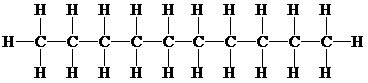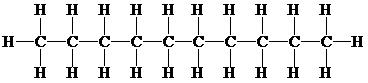# Drawing Structural and Condensed Structural Formulas Give the molecular formula, and draw the structural formula and condensed structural formula for the alkane with 10 carbon atoms.BuyFind

### Chemistry In Focus

7th Edition
Tro + 1 other
Publisher: Cengage Learning,
ISBN: 9781337399692BuyFind

### Chemistry In Focus

7th Edition
Tro + 1 other
Publisher: Cengage Learning,
ISBN: 9781337399692

#### Solutions

Chapter 6, Problem 6.1YT
Textbook Problem

## Drawing Structural and Condensed Structural FormulasGive the molecular formula, and draw the structural formula and condensed structural formula for the alkane with 10 carbon atoms.

Expert Solution
Interpretation Introduction

Interpretation:

The molecular formula, structural formula and condensed structural formula are to be represented for the alkane with 10 carbon atoms.

Concept Introduction:

The organic compounds which consist of only carbon and hydrogen atoms are called hydrocarbons. The atoms in hydrocarbons are linked by single, double, or triple bonds.

The graphic representation of a chemical compound is called its structural formula. It represents the arrangement of atoms.

In a condensed structural formula, the bond between atoms in a compound is represented by the absence of a line.

The chemical formula that represents the total number of atoms arranged in a molecule is known as the molecular formula.

## Answer to Problem 6.1YT

Solution: Molecular formula for an alkane is given as:

C10H22

The structural formula for an alkane contains 10 carbon atoms and can be represented as:The condensed structural formula for an alkane has 10 carbon atoms and can be represented as:

CH3CH2CH2CH2CH2CH2CH2CH2CH2CH3

Or

CH3(CH2)8CH3

### Explanation of Solution

The molecular formula for an alkane with 10 carbons bonded with single bond can be represented as follows:

C10H22

The structural formula for an alkane compound which contains 10 carbon and 22 hydrogen atoms linked together by single bond can be represented as follows:The condensed structural formula for a pentane consists of 10 carbon and 22 hydrogen atoms bonded to each other by single bonding. The name of the compound is decane. The condensed structural formula can be represented as follows:

CH3CH2CH2CH2CH2CH2CH2CH2CH2CH3

Or

CH3(CH2)8CH3

Conclusion

Molecular formula, the structural formula and the condensed structural formula for an alkane has 10 carbon atoms are respectively as below:

C10H22,and

CH3CH2CH2CH2CH2CH2CH2CH2CH2CH3

Or

CH3(CH2)8CH3

### Want to see more full solutions like this?

Subscribe now to access step-by-step solutions to millions of textbook problems written by subject matter experts!

### Want to see more full solutions like this?

Subscribe now to access step-by-step solutions to millions of textbook problems written by subject matter experts!© 2021 bartleby. All Rights Reserved.